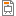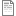# Analytic geometry

Euclidean, spherical, hyperbolic, and projective geometry. Analytic geometry. Vector algebra as the algebraic basis of the analytic version of Euclidean geometry. Vector operations and their properties. Cartesian coordinate system. Combination of points. Equations of lines, planes and circles. Affine transformations and matrices. Complex numbers as another algebraic basis of Euclidean planar geometry. Differentiation and its algebraic representation: Clifford algebras, dual numbers, and automatic differentiation.

Képek:Geometries and algebras - PPTGeometries and algebras - PDFVectors and matrices - CPP headerTransformation with complex numbers - CPPDerivation with Clifford algebra - CPP